# Stamps and albums

Michail has in three albums stored 170 stamps. In the first album, there are 14 more than in the second and in the second is by 1/5 less than in the third. How many stamps are in the first album?

x =  62

### Step-by-step explanation:

170 = x+y+z
x = 14+y
y = z- z/5

x+y+z = 170
x-y = 14
5y-4z = 0

x = 62
y = 48
z = 60

Our linear equations calculator calculates it.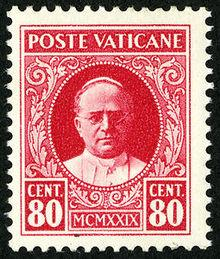Did you find an error or inaccuracy? Feel free to write us. Thank you!Tips to related online calculators
Do you have a linear equation or system of equations and looking for its solution? Or do you have a quadratic equation?

## Related math problems and questions:

• Three friends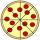Divide 570 euros to three friends so that first will get 50 euros less than the second and third twice more than the first. How many euros will get everyone?
• SchoolsThree schools are attended by 678 pupils. To the first attend 21 students more and to the third 108 fewer students than to second school. How many students attend the schools?
• Three granaries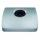Three granaries hold 7,800 metric cent (q) of grain. In the first, 70 tonnes of grain was more than the second and in the third it was 120 tonnes less than in the second. How many grain were in each granary?
• Farm2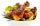The farm bred turkeys, geese, hens, and chickens. Everything is 400. Turkeys and geese a hen are 150. No hen has more than one chicken, but some hens have no chick. Half of them are also a quarter of the chickens. When the turkey was subtracted by 15, we
• Three daysDuring the three days sold in stationery 1490 workbooks. The first day sold about workbooks more than third day. The second day 190 workbooks sold less than third day. How many workbooks sold during each day?
• BoxesTwo hundred boxes have been straightened in three rows. The first row was 13 more than in the second, and in the second was one fifth more than in the third one. How many boxes are in each row?Number 118 divide into two addends, so the first addend is 69 greater than 75% of the second addend.
• Potatoes bags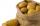I have three bags with 21 kg potatoes. The first bag is 5.5 kg more than in the second bag, and the third is 0.5 kg more than in the second bag. Determine how many kgs of potatoes are in each bag.
• Warehouses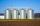In the three warehouses, a total of 70 tons of grain was stored. In the second warehouse was stored 8.5t less and in the third 3.5t more than in the first. How many tons of grain was stored in each warehouse?
• Three workersThe company rewarded three workers CZK 9200, and the money was divided by the work they have done. The first worker to get twice than the second, the second three times more than the third. How much money did each worker receive?
• Buttons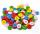Shirt has 6 buttons, blouse has 4 buttons. All buttons are 176. Shirts and blouses are together 36. How many are the shirts and blouses?
• Container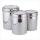First day got off 1/4 water from full container, second day 1/5 of the remaining water. How many percent remain container filled?
• The libraryAnna stored a total of 126 books in three compartments of the library. There are 6 books more in the first compartment than in the second one. The number of books in the second compartment is the arithmetic average of the number of books in the first and
• Tickets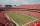The football tournament ticket cost 45 Kc for standing and 120kč for sitting. Sitting spectators was 1/3 more than standing. The organizers collected a total 12 300 Kc. How many seated and standing seats (spectators)?
• MilkThere were 22 liters of milk in three containers. There was 6 liters more in the first container than in the second. After pouring 5 liters from the first container into the third container, the same quantity of milk is in the second and third container.
• Apartments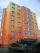Apartment on the first floor was 10% more expensive than the same apartment on the second floor. The difference was 105 Kc annually. Calculate the annual rent of the apartment in the first floor and from apartment on the second floor.
• Pumps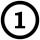First pump flows 16 liters per second into the basin by the second pump 75% of the first and by third pump half more than the second. How long will take fill basin by all three pumps simultaneously volume 15 m3 (cubic meters)?